Note: The other languages of the website are Google-translated. Back to English

## How to use IF function with AND, OR, and NOT in Excel?

In Excel, the IF function is quite useful to check whether a cell meets a condition and return different values based on evaluated results (True and False). And combining the IF function and AND, OR, NOT functions, it will vary conditions to check cells. Here I will introduce how to use IF and AND functions, IF and OR functions, and combine multiple IF functions in Excel.

Supposing we have a sales table as the following screen shot shown, and now we want to evaluate the sale values and group them as "Unordinary", "Low", "Middle", and "High".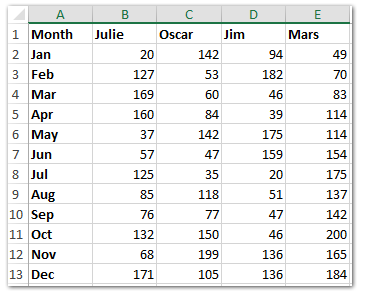And Now copy the row headers and column headers into a blank range (Range A16:E28 in our example) as the following screen shot shown.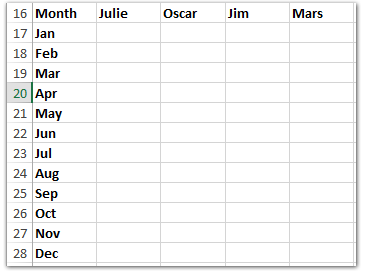#### How to use IF function in Excel

The basic form of IF function in Excel is shown as: =IF(logic_test, value_if true, value_if_false)

In our case we want to check the sale volumes are Low or not. If the value in Cell B2 is equal or less than 80, return the text of "Low", if the value in Cell B2 is bigger than 80, return blank.

Enter the formula =IF(B2<=80,"Low","") into the Cell B17, and then drag the Fill Handle to the Range B17:E28. See screen shot below: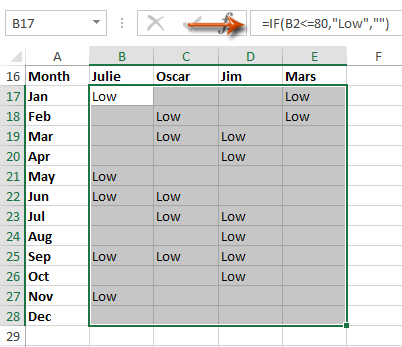#### Easily combine multiple worksheets/workbooks/CSV files into one worksheet/workbook

It may be tedious to combine dozens of sheets from different workbooks into one sheet. But with Kutools for Excel’s Combine (worksheets and workbooks) utility, you can get it done with just several clicks! Full Feature Free Trial 30-day!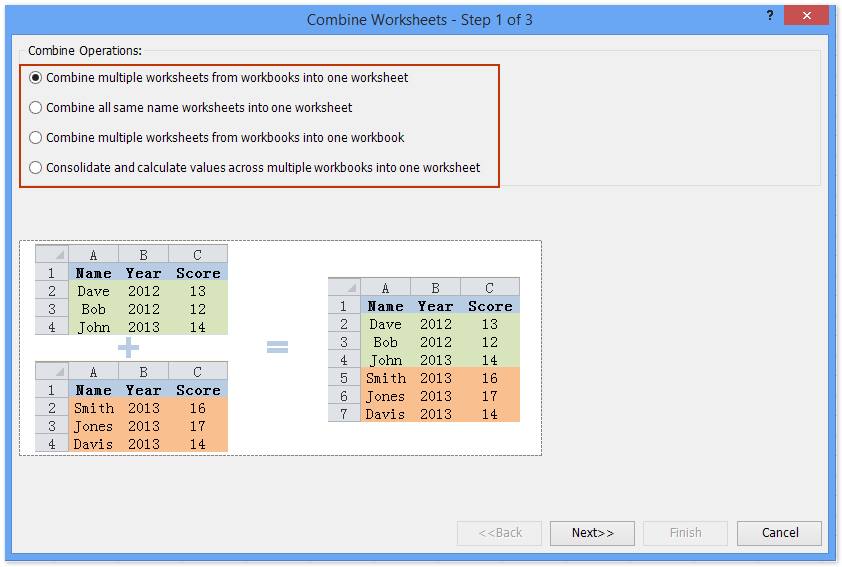#### How to use If function and AND function in Excel

If you want to check if a cell meets several conditions at the same time, you can combine the IF function and AND function in Excel.
The basic form of AND function in Excel is =AND(Logical 1, Logical 2, Logical 3, …, etc.)

Now in our case we want to check a sale volume is more than 80 and less than 160 simultaneously, if yes returns the text of "Middle", and if no returns blank.

Enter the formula =IF(AND(B2>80,B2<160),"Middle","") into Cell B17, and then drag the Fill Handle to the Range B17:E28. See screen shot below: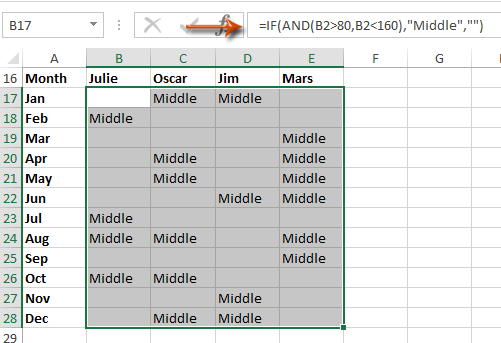#### How to use IF function and OR function in Excel

If you want to check if a cell meets one of multiple conditions, you can combine the IF function and OR function in Excel.

The basic form of OR function in Excel is =OR(Logical 1, Logical 2, Logical 3, …, etc.)

In our example, we want to find out the unordinary sale volumes which are bigger than 180 or less than 20. If the sale volumes are unordinary, return the text of "Unordinary", if no return blank.

Enter the formula =IF(OR(B2>180,B2<20),"Unordinary","") into Cell B17, and then drag the Fill Handle to the Range B17:E28. See screen shot below:#### How to combine multiple IF functions into one formula in Excel

In our example, if you want to evaluate all sale volumes with one formula, you can apply a complex IF function.

If the sale volume is equal or less than 20, return text of "Unordinary"; if the sale volume is equal or less than 80, return text of "Low"; if equal or less than 160, return text of "Middle", and if equal or less than 200, return text of "High".

Enter the formula =IF(B2<=20,"Unordinary",IF(B2<=80, "Low", IF(B2<=160, "Middle", IF(B2<=200, "High", "")))) into Cell B17, and then drag the Fill Handle to the Range B17:E28. See screen shot below: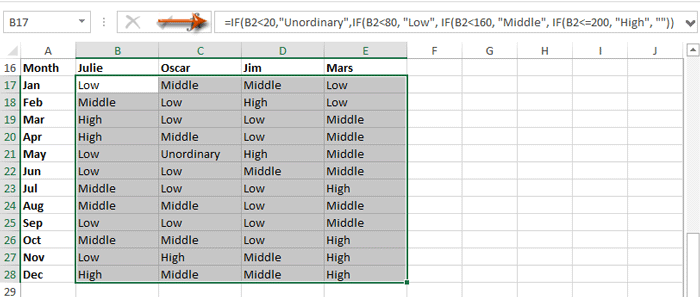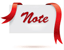Formula is too complicated to remember? Save the formula as an Auto Text entry for reusing with only one click in future! Read more…     Free trial

### The Best Office Productivity Tools

#### Kutools for Excel Solves Most of Your Problems, and Increases Your Productivity by 80%

• Reuse: Quickly insert complex formulas, charts and anything that you have used before; Encrypt Cells with password; Create Mailing List and send emails...
• Super Formula Bar (easily edit multiple lines of text and formula); Reading Layout (easily read and edit large numbers of cells); Paste to Filtered Range...
• Merge Cells/Rows/Columns without losing Data; Split Cells Content; Combine Duplicate Rows/Columns... Prevent Duplicate Cells; Compare Ranges...
• Select Duplicate or Unique Rows; Select Blank Rows (all cells are empty); Super Find and Fuzzy Find in Many Workbooks; Random Select...
• Exact Copy Multiple Cells without changing formula reference; Auto Create References to Multiple Sheets; Insert Bullets, Check Boxes and more...
• Extract Text, Add Text, Remove by Position, Remove Space; Create and Print Paging Subtotals; Convert Between Cells Content and Comments...
• Super Filter (save and apply filter schemes to other sheets); Advanced Sort by month/week/day, frequency and more; Special Filter by bold, italic...
• Combine Workbooks and WorkSheets; Merge Tables based on key columns; Split Data into Multiple Sheets; Batch Convert xls, xlsx and PDF...
• More than 300 powerful features. Supports Office/Excel 2007-2019 and 365. Supports all languages. Easy deploying in your enterprise or organization. Full features 30-day free trial. 60-day money back guarantee.#### Office Tab Brings Tabbed interface to Office, and Make Your Work Much Easier

• Enable tabbed editing and reading in Word, Excel, PowerPoint, Publisher, Access, Visio and Project.
• Open and create multiple documents in new tabs of the same window, rather than in new windows.
• Increases your productivity by 50%, and reduces hundreds of mouse clicks for you every day!No ratings yet. Be the first to rate!
This comment was minimized by the moderator on the site
how do i make this formulas talk to one another: =IF(OR(AND(MID(K2,6,1)="N",(MID(K2,6,1)="C"),(MID(K2,6,1)="H"),(MID(K2,6,1)="I"),(MID(K2,6,1)="B"),(MID(K2,6,1)="F"),(MID(K2,6,1)="L"),(MID(K2,6,1)="M"),(MID(K2,6,1)="P"),(MID(K2,6,1)="R"),(MID(K2,6,1)="P"),ISTEXT(G2)="61"),OR(AND(RIGHT(K2,2)=G2))),"Good","Review") =IF(AND(RIGHT(K2,2)=G2),"Good","Review") or =IF(NOT(OR(AND(MID(K2,6,1)="N",(MID(K2,6,1)="C"),(MID(K2,6,1)="H"),(MID(K2,6,1)="I"),(MID(K2,6,1)="B"),(MID(K2,6,1)="F"),(MID(K2,6,1)="L"),(MID(K2,6,1)="M"),(MID(K2,6,1)="P"),(MID(K2,6,1)="R"),(MID(K2,6,1)="P"),ISTEXT(G2)="61"),(RIGHT(K2,2)=G2))),"Good","Review")
marhsall
This comment was minimized by the moderator on the site
I have charges calculation for eg. charges amount is 0 then next column should be 0. if charges is more than 1 and less than 250 then next column should be 250. If charges is more than 250 then actual charges should come in next column(252)
Raman V
This comment was minimized by the moderator on the site
how to determine the age of the cell ? is this formula right =IF(I2&lt;30,"future payment",AND(IF(I2&gt;30&lt;=45,"30-45days",AND(IF(I2&gt;45&lt;=60,"45-60days",AND(IF(I2&gt;60,"61+days
",0)))))))
pramod
This comment was minimized by the moderator on the site
Hi pramod,
You cannot use I2&gt;30&lt;=45 in formula directly. I have changed the formula for you: =IF(I2&lt;=30,"FUTURE PAYMENT",IF(B2&lt;=45, "30-45 DAYS", IF(B2&lt;=45, "45-60DAYS", IF(B2&lt;=60, "60+DAYS", ""))))
Tang Kelly
This comment was minimized by the moderator on the site
I'm trying to make the formula return 4 possible answer which is (R-regular,A-absent,L-late,OT-overtime). My basis for regular is if the value of the cell is "8". So this is the formula that I made so far =IF(H16&gt;7.9,"OT",IF(H16&lt;7.9,"L",IF(H16=8,"R","A"))). It only returns (OT and L) something is very wrong and i can't figure it out. I'm just new to excel so please help me.
Dan Kinnefer
This comment was minimized by the moderator on the site
Try this: =IF(OR(H16=0,H16=""),"A",IF(H16=8,"R",IF(H16&lt;7.9,"L","OT")))
Anh Nguyen
This comment was minimized by the moderator on the site
I'm trying to make the formula return 4 possible answer which is (R-regular,A-absent,L-late,OT-overtime). My basis for regular is if the value of the cell is "8". So this is the formula that I made so far =IF(H16&gt;7.9,"OT",IF(H16&lt;7.9,"L",IF(H16=8,"R","A"))). It only returns (OT and L) something is very wrong and i can't figure it out. I'm just new to excel so please help me
Dan Kinnefer
This comment was minimized by the moderator on the site
Hi Dan,
Try this formula =IF(H16=8,"R",IF(H16&lt;7.9,"OT","L"))
Tang Kelly
This comment was minimized by the moderator on the site
Im trying to do IF(OR(A1=1;AND(A2=1;A3=1)))
Mirko Tipka
This comment was minimized by the moderator on the site
Hi Mirko,
Thank you for your comment. What calculation result do you want to get? Could you tell me more about your problem?
Tang Kelly
This comment was minimized by the moderator on the site
=IF(OR((I2=(T2+U2),(I2=(U2+V2)),"Closed","OPEN")
HOW TO MAKE THIS RIGHT?
LINCY
This comment was minimized by the moderator on the site
=IF(OR(I2=T2+U2,I2=U2+V2),"CLOSED","OPEN")
Tang Kelly
This comment was minimized by the moderator on the site
Hello, I have a pretty complex formula I am trying to write and it is killing me to admit defeat on this one. So far I have:

=IF(AND(B2="Prudential",OR(C2={"10 Year Term","15 Year Term","20 Year Term","30 Year Term"})),D2*0.75,(IF(C2="UL",D2*0.8,"0")))

and it works just fine. However, I need to add about 37 more companies with their own commission structures to it. I am pretty sure I just need one monster OR statement with a bunch of nested IFs thrown in I just am at my wits end on figuring out exactly where to place them. Any help would be amazing. Thank you!
Dan
This comment was minimized by the moderator on the site
Hi Dan,
Your question is still unclear. you might to explain more by providing a solution for at least 2 companies. However, just help to shorten your OR function a bit. OR(C2={"10","15","20","30"}&amp;" Year Term").
Anh Nguyen
This comment was minimized by the moderator on the site
Hello! I am currently creating a template that will provide me a value depending on the score of the trainee.

I was hoping I can add a value of 5 for any team member who gets a certain quiz %

Here's what I'm thinking.

5= 100%
4= 99.9% to 90%
3= 90% to 85%
2= 85% to 80%
1= &lt;79.99

I hope you guys can help me with the formula
Kim Detera
This comment was minimized by the moderator on the site
Hi Everyone, I need to write a formula for the following:

IF A1 = "BOTTOMS" AND A9 &lt;14, then 14, otherwise A9

OR A1 = "TOPS" AND A9 &lt;5, then 5, otherwise A9
FG
This comment was minimized by the moderator on the site
Hi FG，
You can’t create a formula to meeting one of both your conditions in Excel.
Tang Kelly
This comment was minimized by the moderator on the site
Hi Kelly!

I actually figured out a workaround!

=ROUND((IFERROR((IF(OR(AND(\$I9="BOTTOMS",BL9&gt;=\$BY\$3),AND(\$I9="TOPS",BL9&gt;=\$BY\$4)),BL9,VLOOKUP(\$I9,\$BX\$3:\$BY\$4,2,FALSE))),0)),0)

I9 - populates "BOTTOMS" or "TOPS" dependant on merchandise code entered
BL9 - minimum set defined by store indice
BY9 - minimum set defined by buyer (i.e the 14 units for bottom and and the 5 units for tops)
BX\$3:\$BY\$4 - vlookup to minimum set defined by buyer if if statement is false
FG
This comment was minimized by the moderator on the site
Hi. How can I write this as formula?

If year is equal to current year, add 1.
if year is previous year, add 1 OR start 1.
if year is 2 years prior, add 1 OR start 1.
KJ
This comment was minimized by the moderator on the site
I am trying to write a formula to look at

1. Is the figure either over £1000, or under -£1000

2. Plus is the next cell over 55% or more

so far I have =OR(M4&gt;=1000,M4&lt;=-1000) which pulls in the over or under £1000 plus or minus but am unsure how to add the second criteria presume its an AND(N2&gt;=55%) but when I add it to the formula it doesnt work.
Michelle
This comment was minimized by the moderator on the site
Hi Michelle,
Tang Kelly
This comment was minimized by the moderator on the site
How would I write this into one function?

If D26 and E26 are blank, then C26-L7
If D26 is not blank and E26 is, then D26-L7
If E26 is not blank, then E26-L7
G
This comment was minimized by the moderator on the site
=IF(AND(D26="",E26=""),(C26-L7),IF(AND(D26&lt;&gt;"",E26=""),D26-L7,E26-L7))
Tang Kelly
This comment was minimized by the moderator on the site
III
II
II
II

II
II
II
II

How can I make a formula in excel -If any one value of the column is III the result shall be displayed as III, if any value of the column is II then the result shall be displayed as II if II and III not present it shall be displayed as I
Kiran
This comment was minimized by the moderator on the site
This comment was minimized by the moderator on the site
Hi Haris,
Tang Kelly
This comment was minimized by the moderator on the site
Hello,
What would the equation for the following be?

In cell K2 enter a formula using the IF and OR functions, as well as structured references, to determine if Adam Moriarty can be a group leader.
a. The IF function should first determine if the staff member’s Service Years is greater than 3 OR if the staff member’s college graduate status is “Yes”. Remember to use a structured reference to the Service Years and the College Graduate columns.
b. The function should return the text Yes if a staff member meets one or both of those criteria.
c. The function should return the text No if a staff member meets neither of those criteria.
jessjess
This comment was minimized by the moderator on the site
Hi jessjess,
Thank you for your comment. I am sorry I can’t figure out a proper formula to meeting your conditions. To know more about the IF function, I guess this webpage is helpful.
https://support.office.com/en-us/article/IF-function-69aed7c9-4e8a-4755-a9bc-aa8bbff73be2
Tang Kelly
This comment was minimized by the moderator on the site
Column A has Numerical Values. Column C &amp; D have text.

If 2 or more separate Numerical Values in any row of Column A match then evaluate those Rows in Column C &amp; D for any Text that doesn't match a given Text and count as 1. If Text does match then a value of 0 is assigned.

So if..

A1:A2 = 1

A3:A4 = 2

C1=Dog
C2=Cat
C3=Dog
C4: Horse

D1=Cat
D2=Dog
D3=Horse
D4=Dog

Since A1 &amp; A2 match each other, evaluate C1,C2,D1,D2 and if any cells do not match either "Dog" or "Cat" assign a value of 1. In this example, since all cells contain either "Dog" or "Cat" a value of 0 would be assigned.

Furthermore,

Since A3 &amp; A4 match each other, evaluate C3,C4,D3,D4 and if any cells do not match either "Dog" or "Cat" assign a value of 1. In this example, since cells C4 &amp; D3 contain something other than "Dog" or "Cat" a value of 1 would be assigned.

Cab
This comment was minimized by the moderator on the site
Hi Cab,
To know more about the IF function, I guess this webpage is helpful.
https://support.office.com/en-us/article/IF-function-69aed7c9-4e8a-4755-a9bc-aa8bbff73be2
Tang Kelly
This comment was minimized by the moderator on the site
Hi,

In a row, 3 columns with different value must show a different interpretation in the target cell.

=IF(A3&gt;0,"Welcome",IF(B3&gt;0,"Win",IF(C3&gt;0,"Will","HI")))

Thanks Pinaki
Pinaki Bhattacharyya
This comment was minimized by the moderator on the site
Hi Pinaki,
In your formula, If A3 &gt; 0, both B3 and C3 also might be greater than 0. Similarly, when B3 &gt; 0, both A3 and C3 also might be greater than 0.

Normally, in the If function, you can set =IF(A3&gt;0, "Welcome",””), which means when A3&gt;0, returns “welcome”, while when A3 is not less than 0, returns nothing. In this function, the two condition A3&gt;0 and A3&lt;=0 are exclusive.

In my example =IF(B2&lt;=20,"Unordinary",IF(B2&lt;=80, "Low", IF(B2&lt;=160, "Middle", IF(B2&lt;=200, "High", "")))), The third condition B3&lt;=160 includes the second condition B2&lt;=80, and the second contains the first too.
kelly001
This comment was minimized by the moderator on the site
Hello all,

I am trying to build an IF statement to help with our Service Level calculations. We need one Queue Name to have a different time requirement than all of the other queues. I am trying to figure out how to get the formula to count all Queue Names (Column B) to show they are within our Service Level Agreement (SLA) if they are in the queue for less than 30 seconds. However, for the Queue named TS_DX_CP_ES*, I need it to show it is within SLA if less than 90 seconds.

I currently have the following formula in place and it works for the 90 second rule but if I try to next the IF formula and change the Queue Name and Time Requirement, I get an error.

Formula that works currently =IF(AND([@[Queue Name]]="TS_DX_CP_ES*",[@[Queue time]]&lt;0.00104166666666667),1,0)

Formula that gives a #VALUE! Error - =IF(AND([@[Queue Name]]="TS_DX_CP_ES*",[@[Queue time]]&lt;0.00104166666666667),1,0),IF(AND([@[Queue Name]]="TS_PLU_US*",[@[Queue time]]&lt;0.000358796296296296),1,0)

Matt Odom
This comment was minimized by the moderator on the site
Hello all,

I am trying to build an IF statement to help with our Service Level calculations. We need one Queue Name to have a different time requirement than all of the other queues. I am trying to figure out how to get the formula to count all Queue Names (Column B) to show they are within our Service Level Agreement (SLA) if they are in the queue for less than 30 seconds. However, for the Queue named TS_DX_CP_ES*, I need it to show it is within SLA if less than 90 seconds.

I currently have the following formula in place and it works for the 90 second rule but if I try to next the IF formula and change the Queue Name and Time Requirement, I get an error.

Formula that works currently =IF(AND([@[Queue Name]]="TS_DX_CP_ES*",[@[Queue time]]&lt;0.00104166666666667),1,0)

Formula that gives a #VALUE! Error - =IF(AND([@[Queue Name]]="TS_DX_CP_ES*",[@[Queue time]]&lt;0.00104166666666667),1,0),IF(AND([@[Queue Name]]="TS_PLU_US*",[@[Queue time]]&lt;0.000358796296296296),1,0)
Matt Odom
There are no comments posted here yet